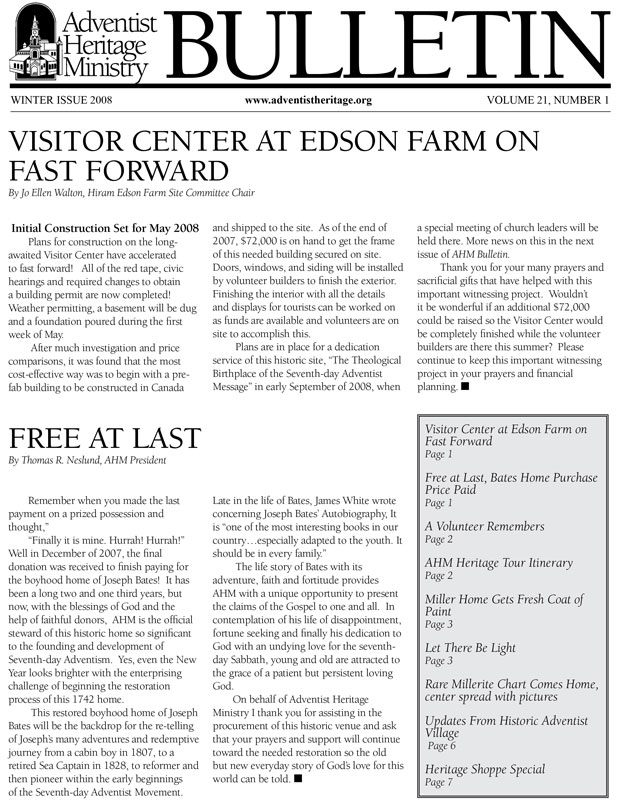#### 2012 (Volume 25)Number 1Number 2Number 3Number 4

#### 2011 (Volume 24)Number 1Number 2Number 3Number 4

#### 2010 (Volume 23)Number 1Number 2Number 3

#### 2009 (Volume 22)Number 1Number 2Number 3Number 4

#### 2008 (Volume 21)Number 1Number 3Number 4Number 5

#### 2007 (Volume 20)Number 1Number 2Number 3

#### 2006 (Volume 19)Number 1Number 2Number 3

#### 2005 (Volume 18)Number 1Number 2Number 3Number 4Number 5

#### 2004 (Volume 17)Number 1Number 2Number 3Number 4

#### 2003 (Volume 16)Number 1Number 2Number 3Number 4Number 5